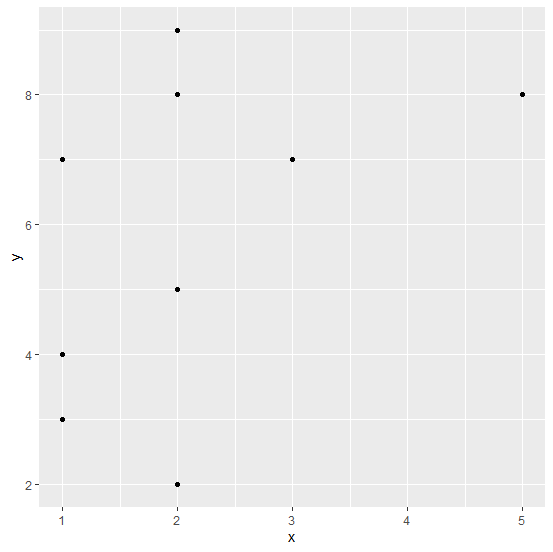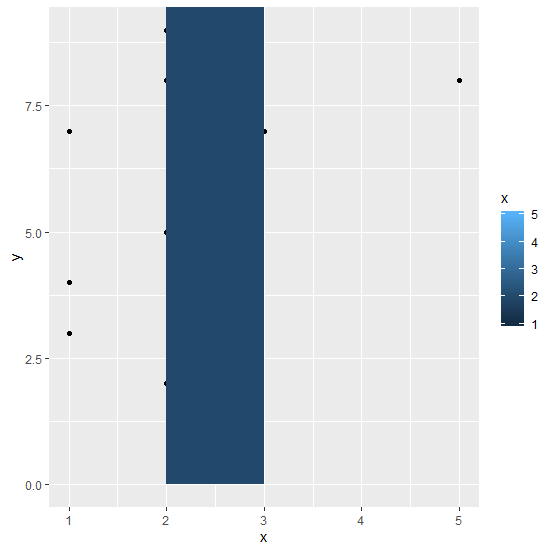# How to create two vertical lines on a plot with shaded area in-between using R?

Sometimes we want to place a vertical rectangle on a plot that has different color as compared to the rest of the plot area. This vertical rectangle is created based on the conditional values of x axis and represent the pivot area or unimportant area depending on the characteristics of the data. These values of x variable are placed as vertical lines on the plot and the area between these lines is shaded. It can be done by using geom_rect function.

## Example

Consider the below data frame −

Live Demo

> x<-rpois(10,2)
> y<-rpois(10,5)
> df<-data.frame(x,y)
> df

## Output

  x y
1 2 9
2 3 7
3 1 3
4 2 2
5 1 4
6 5 8
7 2 8
8 1 4
9 1 7
10 2 5

Creating a scatterplot between x and y −

> library(ggplot2)
> ggplot(df,aes(x,y))+geom_point()

## OutputAdding vertical lines at x=2 and x=3 with shaded area in-between −

> ggplot(df,aes(x,y))+geom_point()+geom_rect(aes(xmin=2,xmax=3,ymin=0,ymax=Inf,fill
=x))

## Output